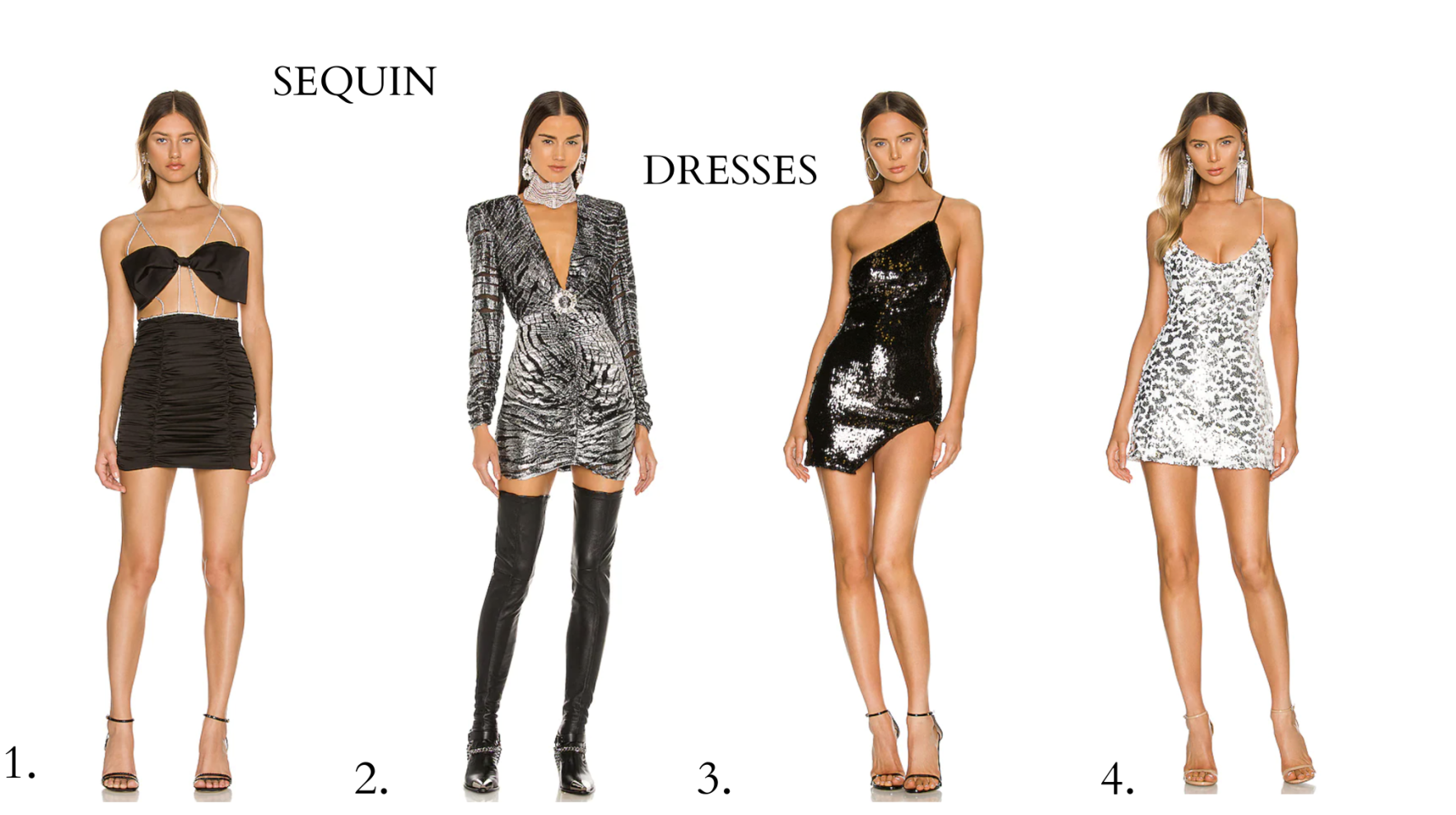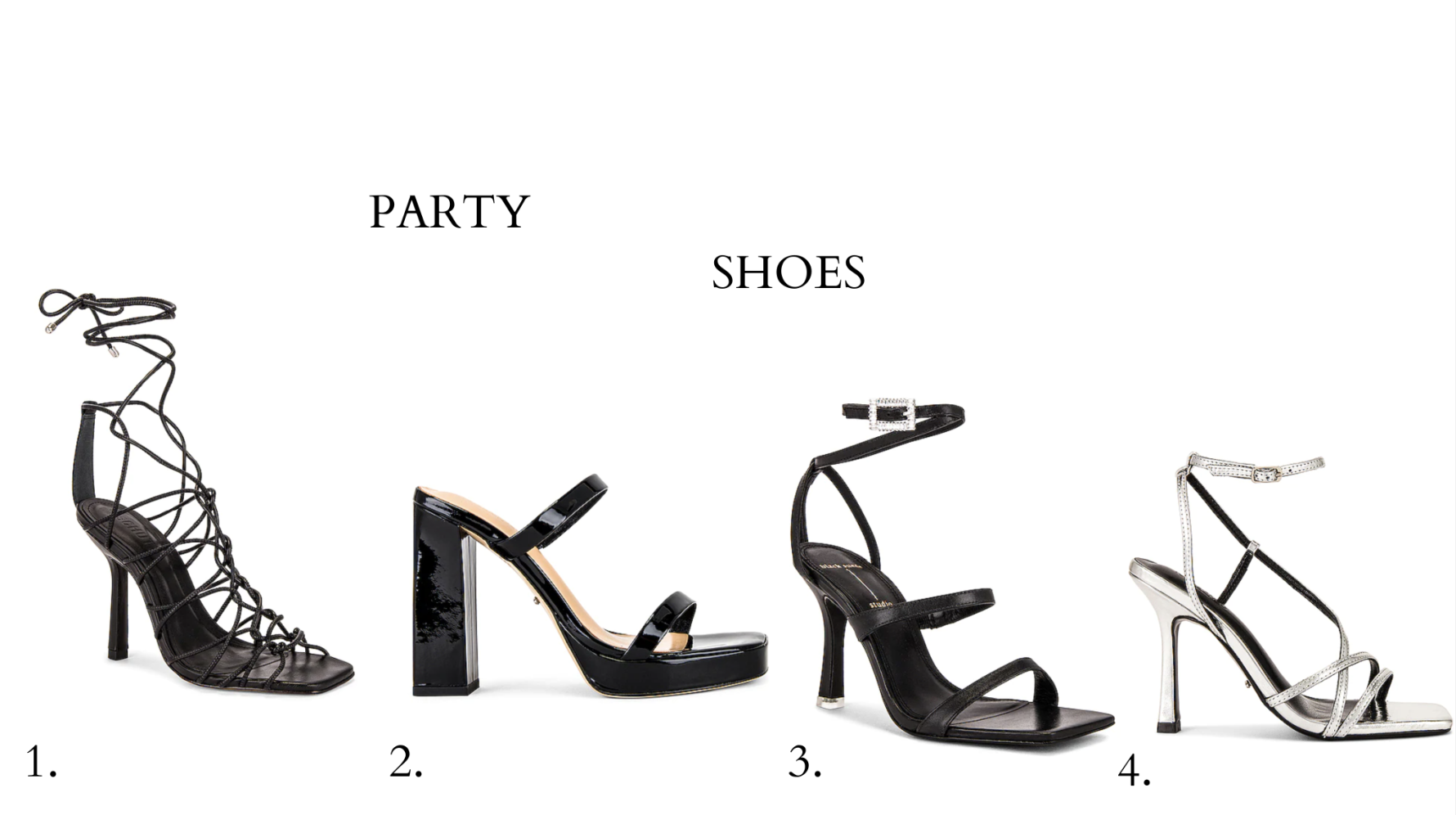`1. HERE / 2. HERE / 3. HERE / 4. HERE``1. HERE / 2. HERE / 3. HERE / 4. HERE``1. HERE / 2. HERE / 3. HERE / 4. HERE``1. HERE / 2. HERE / 3. HERE / 4. HERE`

Can you guess which one I chose?